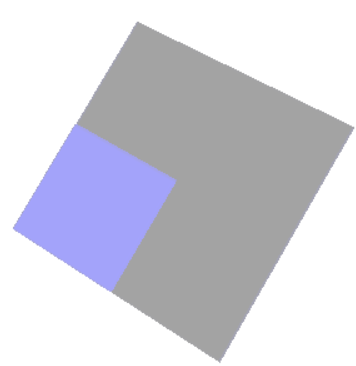# vtkActor.SetColor do not work for vtkContourFilter

I have a binary mask, and I want to display the isoface. The special mask is zero for all pixel except that the intensity is one for two slice, for example

``````
import vtkmodules.all as vtk

img = vtk.vtkImageData()

img.SetDimensions(256, 256, 256)
img.AllocateScalars(vtk.VTK_DOUBLE, 1)

# initial all zero
for i in range(256):
for j in range(256):
for k in range(256):
img.SetScalarComponentFromDouble(i, j, k, 0, 0)

for i in range(64, 192):
for j in range(64, 192):
img.SetScalarComponentFromDouble(i, j, 128, 0, 1)

for i in range(64, 128):
for j in range(64, 128):
img.SetScalarComponentFromDouble(i, j, 129, 0, 1)

``````

The mask is `256x256x256`, and is initialized as zero. There are some pixels are one for `128/129` slice.

Then, I want to compute the isosurface and display it. The code is:

``````
cf = vtk.vtkContourFilter()
cf.SetInputData(img)
cf.ComputeNormalsOn()
cf.SetValue(0, 1)
cf.Update()

mapper = vtk.vtkPolyDataMapper()
mapper.SetInputData(cf.GetOutput())
actor = vtk.vtkActor()
actor.SetMapper(mapper)
actor.GetProperty().SetColor(0, 0, 1) # set to blue color
actor.GetProperty().SetOpacity(0.2)

renderer = vtk.vtkRenderer()
renderer.SetBackground(1, 1, 1)
renWin = vtk.vtkRenderWindow()
iren = vtk.vtkRenderWindowInteractor()
iren.SetRenderWindow(renWin)
iren.Initialize()
iren.Start()

``````

And the result is:I set the color to blue by `actor.GetProperty().SetColor(0, 0, 1)`, but there is some gray part. What’s wrong with my code? How to set the color to blue?

Any suggestion is appreciated~~~

``````mapper.ScalarVisibilityOff()
``````

If a data set has scalars, then vtkMapper will display the scalars and ignore the property color. The VTK contour and clip filters create scalars, so it is necessary to use `SetScalarVisibilityOff()` to display their output with a solid color.

Unfortunately, `mapper.ScalarVisibilityOff()` do not work, and the shown figure do not change.

Actually, `vtk.vtkContourFilter.SetValue(0, 1)` only extract one surface, thus there should by only one color. But, the resulted figure shows two color. I think the result should not be the `ScalarVisibility`.

It seems to be a problem with the normals. Where the mask is only once slice, the normals have a value of (0,0,0).

To generate a contour between values of 0 and 1, a contour value of 0.5 might work better, since it is halfway between the two values:

``````cf.SetValue(0, 0.5)
``````
1 Like

It works. Thanks~~~